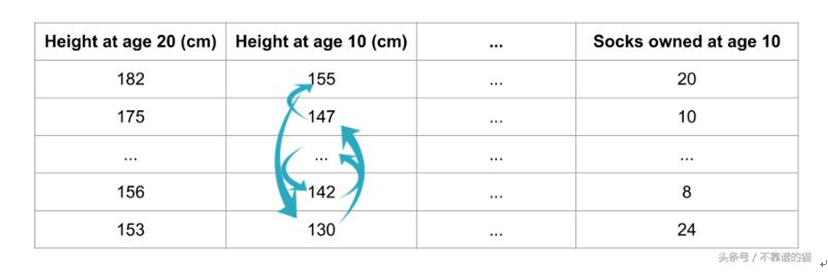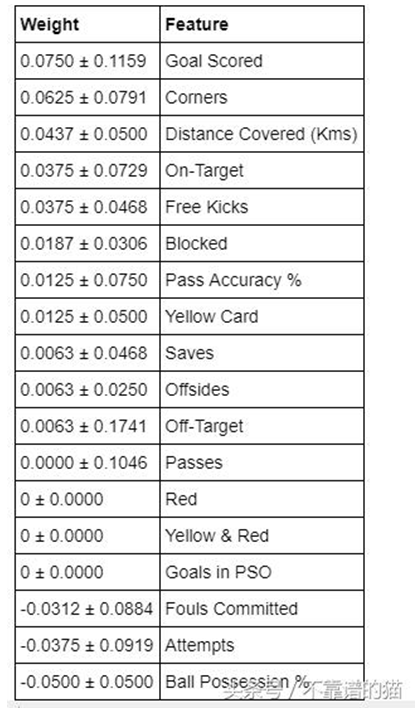# 哪些特征对我的机器学习模型影响最大？

• 快速计算

• 易于使用和理解

• 它具有我们想要的特征重要性度量的属性。

# 它是如何工作的 ？Fig.1 Random shuffle of 'Height at age 10(cm)' col

# Python 代码示例

```
import numpy as np

import pandas as pd

from sklearn.model_selection import train_test_split

from sklearn.ensemble import RandomForestClassifier

y = df['Man of the Match']=='Yes'

features = [i for i in df.columns if df[i].dtype in [np.int64]]

X = df[features]

train_X, val_X, train_y, val_y = train_test_split(X, y, random_state=1)

model = RandomForestClassifier(random_state=0).fit(train_X, train_y)

```

```
import eli5

from eli5.sklearn import PermutationImportance

perm = PermutationImportance(model, random_state=1).fit(val_X, val_y)

eli5.show_weights(perm, feature_names=val_X.columns.tolist())

```• 博文量
2302
• 访问量
3466149